By Ronnie Brown, Professor Emeritus, University of Wales Bangor.

Contents. (The paper is split into 3 web pages for browsing)

 First page 1This web version of the paper includes images from the popularisation web site (click on the image) and accompanying CD. Contact the author for more information and to obtain a copy of the CD. For mathematicians: "From groups to groupoids: a brief survey" (ref ) also by the author. BOOK: Topology and Groupoids. R. Brown 2006 3rd edition. Order online. 2 4 Second page 7 9 This page. VI. Towards Higher Dimensions 15 18 22 IX.Implications. References. 24 PDF.PDF version 24pp, 700KB OPEN/DOWNLOAD

VI Towards higher dimensions

At last I can move into higher dimensions, and explain how we get a notion which is not exactly a higher dimensional group, but does perform the same intended role. The next stage in the history is 1932, when the symmetry groups and the Poincaré group were standard methods, with applications right across mathematics, and also in science. In that year the Czech mathematician, E. Cech submitted a paper on "Higher homotopy groups" (the term homotopy refers to a particular branch of Topology concerning holes in spaces) to the Proceedings of the International Congress of Mathematicians at Zurich. Instead of mapping loops to a space, his idea was to map spheres. One can also map squares, or their higher dimensional analogue. Cech used one of the dimensions to define the group structure, in a similar way to what I showed for loops. However, Alexandroff and Hopf, the kings of Topology of the time, showed, by using one of the extra dimensions, that Cech's groups were always commutative: .
 xy =yxFig 45.

Here is the key idea of why this is so. In two dimensional group theory, based on squares rather than paths, one may formulate the product of two squares as given by any of the diagrams in Fig 45. That is, in two dimensions there is more room to move around, and this leads to the commutativity rule. The 2-dimensional theory is thus simpler than the 1-dimensional theory (which seems absurd!).

On this ground, and because it was felt that the groups must be the same as the already known homology groups, Alexandroff and Hopf persuaded Cech to withdraw his paper and only a small paragraph appeared in the Proceedings of the Congress. Three years later, however, a Dutch mathematician, W. Hurewicz, published four Notes explaining the main properties of these higher homotopy groups, but without referring to Cech's paper, so they have come to be known as the Hurewicz homotopy groups. These higher homotopy groups became very important concepts, with many people working on them, despite or even because of the difficulty of calculating them for some standard spaces. Both Alexandroff and Hopf later admitted their mistake over Cech's paper. In the 1960s, when higher homotopy groups, despite their being commutative, had become a fundamental tool in topology and geometry, Hopf told E. Dyer that it showed the error of people regarding themselves as so great they are able to know what shall be the future.

This commutativity of higher homotopy groups put paid to the hope of a reasonable "higher dimensional group theory", appropriate for work in two or more dimensions, and more subtle than ordinary group theory. A range of other methods were developed to deal with higher homotopy groups, and to analyse higher dimensional spaces. But any attempt to model directly the 1-dimensional theory in higher dimensions was regarded as a failure to recognise a standard, and fairly simple, fallacy.

VI Groupoids: a wider structure than groups.

It was fortunate that, through being led into considering the notion of groupoid, a generalisation of abstract group which had been around since 1926, I and colleagues were able to open the door into a new theory. In 1965 I was writing a text on Topology, and was busy explaining the theory of the Poincaré group, and its calculation by the Van Kampen Theorem. I came across a 1964 paper of Philip Higgins using groupoids in group theory. This suggested turning my exposition away from the standard format, and to put the emphasis on the known Poincaré groupoid.

Groups arise from composing loops as we showed earlier. But in making a journey, you do not always return to the starting point. You might want to move from A to B, and then from B to D. In fact you can continue a journey a from A to B with a journey b from C to D if and only if B = C. (Obvious!) So, we make this intuition into a mathematical structure. The name for this new and more flexible analogue of a group is groupoid . Its rules, its axioms, are as for groups, with the difference that the composite or product ab is not always defined.

The best way to see this, which emphasises the geometric character of the definition, is to say that for a groupoid we have arrows each with two vertices (fig 46), the source (on the left) and the target (on the right) and that two arrows can be composed to give a product ab if and only if the target of a is the same as the source of b (fig 47).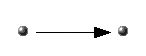Fig 46. Fig 47.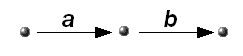One imposes the geometrically obvious notions of associativity, left and right identities, and inverses. Thus a groupoid is often thought of as a group with many identities, and the reason why this is possible is that the product ab is not always defined.

We now know that this apparently anodyne relaxation of the rules has profound consequences. The reason seems to be that the algebraic structure of product is here linked to a geometric structure, namely that of arrows with source and target, and which mathematicians call a directed graph. Thus a groupoid can be thought of as having structure in dimensions 0 (namely the vertices) and 1 (namely the arrows). The linking of these two structures allows for groupoids to be more convenient for modelling geometric phenomena than are groups alone . This came to be the main message of the second half of my 1968 Topology book .

We can use this extension from groups to groupoids to study symmetry. Recall that the symmetry group of the square has 8 elements. Now the operation called x rotates through 90° , i.e. takes whatever is in that position and moves it appropriately. Thus the group structure does not fully describe the situation. The full description involves all the eight positions of the square and all the ways of moving between them, a total of 64 elements. These movements, and their compositions, fit together into an abstract groupoid, which gives a fuller description of the symmetry of the square than just the usual group of symmetries. People who work with groupoids recognise that groupoids allow for a fuller and more flexible approach to symmetry than do groups alone.

This notion of groupoid occurs all over the place. In the early part of this century, the mathematician H. Brandt was led to groupoids through his work over many years on generalising work on number theory due to Gauss, one of the great mathematicians of all time. Brandt's paper on groupoids appeared in 1926, and the idea of groupoid was for a long time used occasionally in algebra and topology, not as a necessary or fundamental concept, but with curiosity at the way it sometimes permitted an elegant treatment. This elegance should have been taken as a clear sign that the concept was an appropriate generalisation of groups in many geometrical applications. This recognition is still patchy.

There is now a large literature on groupoids (see ), with applications ranging across mathematics, from topology to algebra, and functional analysis. There are applications in physics. Indeed, Connes  has recently pointed out that Heisenberg in 1925 realised that the Ritz-Rydberg law for the spectrum of hydrogen is a groupoid law rather than a group law. This led Heisenberg to consider an algebra of observables for this groupoid law, the so called convolution algebra for the groupoid, and it was only later that Max Born noticed that this algebra was the same as the algebra of matrices, which was very familiar in mathematics. So the groupoid interpretation vanished from the physics scene. Only now has it been revived under the influence of Connes and others.

VII. Higher dimensional groupoids

The main point I want to convey is that the simple algebra of composing arrows that we saw for a groupoid has an easy extension to higher dimensions, which was formulated by Ehresmann in the 1950s. He called it a double category, of which a special case is a double groupoid, though he had rather formal reasons for discussing them. The algebra which results may be called an algebra of squares. There are two interesting points here. One was that having convinced myself that groupoids were the appropriate tool in dimension 1, and having been educated in higher homotopy groups, it was forced on me to try to find higher homotopy groupoids, since it would have been unrealistic to suppose that groupoids were useful in dimension 1 and totally useless in higher dimensions. A second point was that the groupoid Van Kampen Theorem gave complete information in a way that seemed anomalous according to the current methods in topology. Thus there was a possibility that a higher dimensional version of the Van Kampen Theorem would open out new methods and allow for complete calculations unobtainable by other methods. The resulting theory thus fits into Kuhn's notion of scientific revolution, in terms of the analysis of an anomaly. It also fits in terms of the scientific opposition to the possibility or utility of such a theory.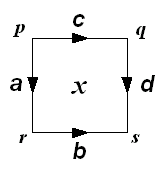Fig 48. Labelled and arrowed square.Fig 49. composition horizontally.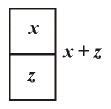Fig 50. composition vertically.Fig 51. Associativity. The idea of double groupoids was and is very simple. The basic objects are squares each with four arrows as its edges and with four vertices. The arrows are to have a product forming a groupoid. Further, the squares are to have two compositions in the two directions as shown (figs 48, 49). It is convenient to label these xy and x + z . The crucial point is that xy is defined if and only the right hand edge of x coincides with the left hand edge of y, and that x + z is defined if and only if the bottom edge of x coincides with the top edge of z. This is exactly what one expects from the geometry. The geometry also prescribes what should be the bounding edges of xy and x + z . One has to insist that all these product and sum structures are groupoids. There is nothing clever in this. It is simply following out the basic assumption that products or compositions are defined under geometric conditions. The reason why thus was not done earlier was a general climate of opinion in which products and compositions of this kind were not taken seriously. Finally, there is one key rule to do with four squares, a kind of associativity, which says that there is only one way of composing a diagram of four squares. In terms of the notation we have used, this says that (xy)+(zw) = (x+z)(y+w), which rather confirms the view that the 2-dimensional picture is easier to understand than the linear formula.

A consequence of this so called "interchange law" is that there is only one way of forming a multiple composition such as that given right at the beginning in Fig 4: you may compose rows first, and then columns, or the other way round, or you can form rectangular blocks and compose each block individually before forming the final composition. This sounds simple. However, sometimes the results of such recalculations of 2-dimensional formulae are startling.

I gave earlier an argument which seemed to dash any hopes of a "higher dimensional group theory", since a "2-dimensional group" is simply a commutative group. The reason why this argument does not work for the new situation is that the squares are tagged together, and cannot move around freely as previously. This shows the difference between "products always defined" and "products defined under geometric conditions". These "double groupoids" are in a real sense "more non commutative" than are groups, and it turns out, in an extraordinary turn of fortune, that double groupoids are nicely tuned to capture the structure of 2-dimensional topology! Thus Alexandroff and Hopf are in a sense vindicated, in that the higher homotopy groups are not the "correct" structure to capture 2-dimensional topology. The "correct" objects are higher homotopy groupoids. The implications of this will take a long time to assimilate.

In order to give more of the flavour of this 2-dimensional algebra, I would like to go go back to the simple aspect, namely the role of identities, which, as I emphasised earlier, are an essential feature of the theories of groups, and of groupoids. In the 2-dimensional theory, any square has four identities, expressing that in dimension 2, there are four ways to stay still, parcelled up into the two directions you can compose squares. The edge arrows of these "stationary" squares look like Fig 52:
 Figs 52a,b. Identities for squareswhere p, q and r denote vertices, and x and y denote arrows and 1p , 1q , 1r are the identities. This is a rather cumbersome notation, and so we abbreviate these as shown where the lines denote identity arrows. Two of the rules for these 2-dimensional identities can then be written in the form:
 a= a, a += a

But there is something else you can do in two dimensions, not allowed in dimension 1, namely make a turn, to the left or the right! This is expressed for example by a square of the form on the left below, which, in analogy with our notation for identities, we write this as on the right of the =.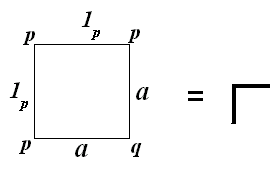Fig 53. In fact we have four squares of this type, written (fig 54):We called these new elements connections, because of a relation with another part of geometry, namely parallel transport, in which the term connection occurs . Contrary to what happens with identities, if you compose any of these connections with a given square, then you usually get a new square! To control this, you need laws on the connections. To see what these laws should be, it is useful to think of a connection as allowing for turning left or right, a possibility in dimension 2 but not in dimension 1. It seems reasonable that if you turn left and then right, or right and then left, then you are pointed in the same direction. This is expressed in terms of our strange symbols by the rules: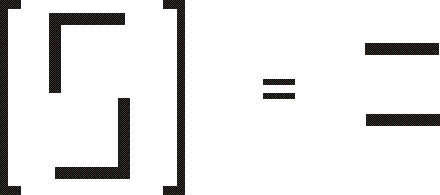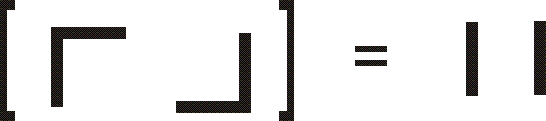This rule is in itself not quite enough for the theory. The law one really needs is called the transport law, where the left hand side might be thought of as turning left with your arm stretched out: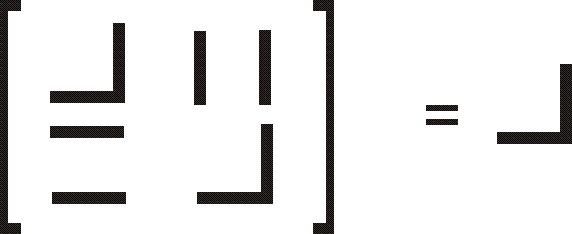Example: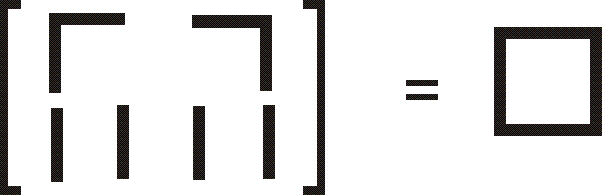A consequence of this law, and the groupoid rules, is for example, the equation on the right.

So we have a new kind of "hieroglyphic" algebra. Notice that these are calculations in dimension two. Two and higher dimensional algebra really is new and has its own concepts, which just do not appear in the easy case of dimension 1, since 1-dimensional geometry, and hence 1-dimensional algebra, is quite restricted (even boring!). The world of higher dimensional algebra is only now beginning to be explored with a strong sense of urgency and competition, as the applications, for example to physics, knot theory and computer science, are emerging.

Our new "connections" also solved one of the questions with which we started: what does it mean for one face of a cube to be a composition of the others? Since we have considered only double groupoids, we need to reduce the problem to two rather than three dimensions. There is a standard way of doing this. Take the cube, remove one face and fold the rest flat. This gives a picture as fig 55: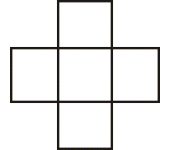Fig 55. Boundary of a cube folded flat Fig 56. Composition of all but one faces of a cube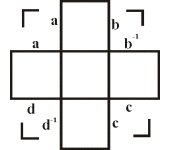There seems to be no way of composing this because of the holes at the corners. However, when I fold the cube flat, I have duplicated the vertical edges: so the adjacent edges of each of the holes are either the same or opposite. I can now use the connections determined by these edges, and give the rule that our remaining face shall be the composition fig 56.

This is just the start of higher dimensional algebra, and the ideas above, which may seem simple, are the key tools in the proof of the the Generalised Van Kampen Theorem, due to Philip Higgins and I in dimension 2 in 1974-5. The other key input was our definition of higher homotopy groupoid, nine years after the first suggestion that these objects should exist, and 42 years after Cech's definition of higher homotopy groups. This led to the first in a series of ten joint papers, the last recently published. The Van Kampen Theorem was extended by us to all dimensions in 1977 . The most difficult algebraic part is to transcribe into dimensions greater than two the ideas just described, but without getting lost in a maze of formulae. To this end, one has to avoid the above folding flat process, by using a key idea found by my research student, Keith Dakin, in 1975, namely the idea of a thin cube. This, magically, by using dimension n , enables the composition of many (n -1)-dimensional formulae, without writing down any formulae! This illustrates how a piece of mathematics can become simpler to understand in a more general framework.

A characteristic of the theory and applications is the tradeoff between the linear and the non-linear approach. The non-linear approach is the right one for the theory, and for the formulation and proofs of theorems. The linear approach is best for calculations. The translation from an obvious n -dimensional result to a linear result has yielded results to which the mathematics leads you directly, indeed they almost pop out of the page, but which, externally to the theory, are highly non-trivial. For example, when J.-L. Loday brought to the ideas developed with Higgins and myself his new models of homotopy theory, there resulted a more powerful Generalised Van Kampen Theorem . This led to an entirely new construction in group theory, and so yielded calculations in topology which could not previously be formulated, let alone proved. This construction suggested analogous constructions for other algebraic situations.

Another pursuit is that of higher order symmetry. We know that groups, and groupoids, are involved with symmetry. For groupoids the application is to what might be called variable symmetry, namely the internal symmetries of a family of objects rather than of just one object, as in the case of groups. However, higher dimensional versions of groupoids, and so of groups, exist, and have remarkable applications. It seems reasonable to suppose that there is some notion of higher order symmetry of which they are the mathematical model. Progress has been made, up to dimension 3. It would be good if these ideas were found to have physical applications, for example to fundamental particle physics, where group theory and symmetry plays such a vital role.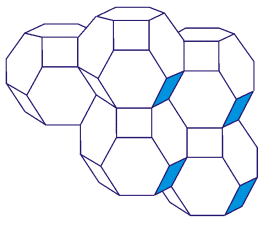Fig 57. Permutahedra

I have emphasised the algebra of squares and cubes, since for these objects it is easiest to see how the compositions or products work, and they give, for that reason, the easiest context in which to formulate and prove theorems. The overall aim is more ambitious, namely a general algebra of compositions, or products, of geometric objects. For example, one would like to be able to formulate what it means to compose, or form a product of, the permutahedra which form the elements of Fig 57.

The picture here is taken from a book on homotopy theory . But the same picture is well known in chemistry as the structure of Zeolite! This shows the remarkable force of geometry in science. In order to form a product of the elements or pieces shown in Fig 57, there are a number of conceptual problems to solve, of the following type:

1. Define precisely the pieces of which you wish to form the product.
2.  Give the precise conditions under which the product can be formed.
3.  Under these conditions, explain how the product is defined, or computed.
4.  Give all the laws for the product.

Of all these, it seems likely that the last is the most difficult.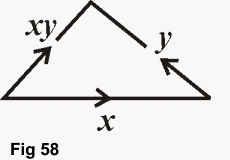One solution to a range of such problems was given by David Jones in his thesis. Keith Dakin's idea of thin elements is crucial. The main point is to think of forming a product xy as constructing a triangle of the following form which might be thought of as representing a computation of this product. Laws for this product are then imposed by higher dimensional figures. Thus in this mathematical structure, geometry and algebra are inextricably linked.

The emphasis on these higher dimensional products or compositions reflects my interest in Generalised Van Kampen Theorems. The intent of such theorems is to calculate an algebraic model of a big space from the algebraic models of small parts of the space. Thus the theorem is of the type common in mathematics, and indeed in practical life, of giving procedures for relating big objects to the small standard pieces from which they are constructed. This is one reason why I feel that this type of algebra is liable to be of wide significance. The methodological aspect is that the Van Kampen Theorems require, in order to be formulated, an algebra in which compositions are of a geometric type reflecting the geometrical properties of the spaces. In particular, the algebraic objects have to have structure in a range of dimensions, since gluing in low dimensions can have strong higher dimensional implications.

You will wish to know how widespread is the knowledge and application of this theory, and where is it likely to lead. There has been a development of similar types of algebra in many places, with different aims, and with an emphasis on the theory of categories. A category is like a groupoid, except that the requirement for inverses is dropped. So categories should be useful for applications to irreversible processes, and this has been amply verified, for example in applications to computer science. The theory of categories was originally invented by Eilenberg and Mac Lane in 1945, as a way of describing some of the formal properties of mathematical theories and structures. Category Theory involves an extended analysis and development of the implications of the arrow notation for functions, f :X â†’ Y, which came into common use in mathematics in the 1940s.

In the hands of many people, but particularly the most extraordinary mathematician of the twentieth century, Alexandre Grothendieck, Category Theory has become a tool for the invention of new languages to analyse mathematical structures and their relationships, to describe new and appropriate formulations of problems, and hence to solve classical problems. Practical tools from category theory have been used in number theory, knot theory, theoretical physics (quantum groups), computer science, and the theory of development in biology.

The theory of multiple categories is now undergoing extensive development. It is in multiple category theory, and so also multiple groupoid theory, that the problem of multiple compositions becomes a central issue. It has been handled in various ways, for example through a theory of "pastings" developed by the Australian school. The problems for multiple categories seem to be considerably more difficult than those for multiple groupoids.

VII Implications

Where are these ideas going to go? How important are they?

My own style of work runs parallel to, and interacts with, a general progress in multiple categories. This has recently had a surge of activity, with applications in several branches of mathematics, for example knot theory and low dimensional topology, and also in physics, particularly quantum groups. The ideas have been shown to be related to the formulation of a quantum field theory, and to subtle relations between knot theory and physics explained for example by Sir Michael Atiyah in .

Workers in computer science are also interested in multiple categories, for example in the theory of rewriting, and for investigations of concurrency. The idea in the first case is that computations are thought of as paths. There are concepts of changing one computation into another, a kind of deformation. This leads to a higher dimensional theory. In the second case, two concurrent processes are thought of as squares, which can develop in the two directions. Thus one approach to concurrency leads to a cubical approach.

One can speculate that the modelling of neurological processes requires structures more complex than the "linear" ones so widely studied in mathematics, and that algebraic objects whose definition depends heavily on geometry could be appropriate for such connectionist modelling. The story of these developments exemplifies the progress of mathematics. Here are the chain of events. Gauss studied the problem of representing a number in a particular form (in fact, x2 +2xy +y2) involving two variables (x and y ). Through this he was led to the structure of commutative group. The corresponding problem for four variables finally led Brandt, about a century later, to the notion of groupoid. This notion knocked around for a number of years, and was taken up by various mathematicians for different purposes, sometimes reinventing the theory from scratch.

In 1965, I showed groupoids could be used not only for theory but also for calculation in topology. This led to the idea of developing the theory and applications of higher dimensional groupoids, using as a basis ideas of multiple category theory developed by Ehresmann for formal reasons. The change of paradigm, from groups to groupoids, was essential for the higher dimensional theory, which now has strong applications in pure mathematics and tremendous prospects. It is reasonable to suppose that higher dimensional algebra will come to bear to ordinary algebra a relation similar to the current relation of many variable calculus to one variable calculus. So the study of abstract structures, even in situations divorced from apparent "real-life" applications, has led to scientific advances and extraordinary new analogies.

It is a pleasure in this to thank all the colleagues and students who have listened to the speculations, seen what needed to be done, and have joined in getting this amazing machine up and working. The intention in this address was to show a new discipline in mathematics still in a new state, with no texts, but with a strong hold on the imagination. The intuition from which it is forged has strong links across science. But the mathematician's search for form and structure also links with the work of the artist and poet. So I hope you will like this quotation from A Midsummer's Nights Dream, since it seems to me to work also for the mathematician:

"The poet's eye, in a fine frenzy, rolling,
Doth glance from heaven to earth, from earth to heaven,
And as imagination bodies forth
The forms of things unknown, the poet's pen
Turns them to shapes, and gives to airy nothing
A local habitation and a name."

REFERENCES

1. Atiyah, Sir Michael F, The Geometry and Physics of Knots, Proc. Royal Institution, 62 (1990) 212-225.
2.  Baues, H, Geometry of loop spaces and the cobar construction, Mem. American Math. Soc., 25 (230) (1980).
3.  Brehm, U., How to build minimal polyhedral models of the Boy Surface, Math. Intell., 12 (4) 1990, 51-56.
4.  Brown, R., From groups to groupoids: a brief survey, Bull. London Math. Soc., 19 (1987) 113-134.
5.  Brown, R., Elements of modern topology, McGraw Hill, 1968; Topology: a geometric account of general topology, homotopy types and the fundamental groupoid, Ellis Horwood, 1988. Revised and extended as Topology and Groupoids, Booksurge, 2006.
6.  Brown, R., Some problems in non-abelian homological and homotopical algebra, in Homotopy theory and related topics: Proceedings Kinosaki, 1988, ed M. Mimura, Springer Lect. Notes in Math. 1418 (1990) 105-129.
7.  Brown, R. and Higgins, P.J., Colimits of relative homotopy groups, J Pure Appl. Algebra, J Pure Appl. Algebra, 22 (1981) 11-41.
8.  Brown, R. and Loday, J.-L., Van Kampen Theorems for diagrams of spaces, Topology, 26 (1987) 311-335.
9.  Brown, R. and Spencer, C. B., Double groupoids and crossed modules, Cah. Top. Géom. Diff., 17 (1976) 343-362.
10.  Connes, A., Geometrie non-commutative, InterEditions, Paris, (1990).
11.  Ferguson, H., Two theorems, two sculptures, two posters, American Math. Month. 97 (1990) 589-610.
12. Dirac, P.A.M., The origin of quantum field theory, in Laurie M. Brown, Lillian Hoddeson editors, The birth of particle physics,   Cambridge University Press, New York :1983.
13.  Kaufmann, L., Knots and physics, World Scientific, 1990.
14.  Robertson, S.A., Polytopes and , symmetry, London Mathematical Society Lecture Notes No 90, Cambridge University Press, 1984.
15.  Robinson, J., Symbolic sculptures and tapestries, Edition Limitée, Geneva, 1992.
16.  Wigner, E.P., The Unreasonable Effectiveness of Mathematics in the Natural Sciences, Comm. in Pure Appl. Math. (1960), reprinted in Symmetries and reflections: scientific essays of Eugene P. Wigner, Bloomington Indiana University Press (1967).Top : Table of Contents.Previous page: Space, Motion...FirstPage: Introduction, Symmetry, ...Home

View My Stats